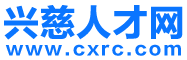• 登录 | 注册• 不限
• 普工技工
• 生产研发
• 文职文员
• 行政人事
• 会计财务
• 物流贸易
• 质控安防
• 电子电气
• 市场销售
• 建筑装修
• 酒店餐饮
• 百货零售
• 客户服务
• 家政保洁
• 保安司机
• 淘宝电商
• 汽车服务
• 教育培训
• 高级管理
• 娱乐休闲
• 医疗保健
• 旅游健身
• 物业管理
• 编辑印刷
• 设计包装
• 机械仪表
• 轻工工艺
• 网络通信
• 化工制药
• 广告会展
• 能源环保
• 美容美发
• 摄影影视
• 房产中介
• 咨询顾问
• 翻译法律拍卖
• 金融保险
• 农林牧渔
• 其他分类

• 不限
• 平面设计
• 三维动画设计
• 多媒体设计与制作
• 产品包装设计
• 室内(外)装修/装潢设计
• 工艺品设计
• 工业产品设计
• 纺织/服饰(装)设计
• 形象设计
• 玩具设计
• 珠宝设计
• 文案/媒体策划
• 广告设计/创意策划
• 家具设计
• 模具设计
• 淘宝/天猫运营
• 美编/美术设计
• 服装设计
• 家具/居家用品设计
• 美术指导
• 店面/陈列/展览设计
• 工艺设计
• 多媒体设计
• 动画设计
• 设计包装其他相关职位

• 不限
• 慈溪市
• 浒山街道
• 白沙路街道
• 宗汉街道
• 坎墩街道
• 古塘街道
• 杭州湾新区
• 横河镇
• 匡堰镇
• 崇寿镇
• 观海卫镇
• 龙山镇
• 周巷镇
• 长河镇
• 庵东镇
• 逍林镇
• 胜山镇
• 新浦镇
• 附海镇
• 桥头镇
• 掌起镇
• 余姚市
• 外地

• 不限
• 今天
• 三天内
• 一周内
• 一个月内
• 三个月内

• 不限
• 全职
• 兼职

• 不限
• 1000元以下
• 1000-2000元
• 2000-3000元
• 3000-5000元
• 5000-8000元
• 8000-1.2万元
• 1.2万-2万元
• 2万元以上
• 面议